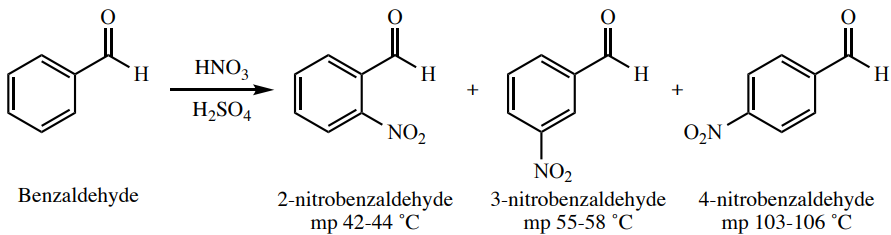# 6.1E: Mixed Melting Points

•• Contributed by Lisa Nichols
• Professor (Chemistry) at Butte College

As previously discussed, there are a large number of compounds that have coincidentally identical melting points. Therefore, caution should be used in identifying a compound based solely on matching the literature melting point. However, mixed melting points offer an ability to almost certainly identify an unknown compound.

Imagine that the nitration of benzaldehyde (Figure 6.17), produces a solid that is determined to have a melting point of $$54$$-$$57^\text{o} \text{C}$$. This solid would be assumed to be 3-nitrobenzaldehyde due to the proximity of the experimental melting point to the literature melting point.Figure 6.17: Nitration of benzaldehyde. The melting point data is from the Aldrich Chemical Catalog.

Although the product likely is as identified, if a pure sample of 3-nitrobenzaldehyde is available, there is a possibility of more strongly identifying the product. A mixed melting point can be taken, by measuring the melting point of a sample composed of roughly equal volumes of the unknown product and of known 3-nitrobenzaldehyde (ground together well with a mortar and pestle, as in Figure 6.18a). If the product is indeed 3-nitrobenzaldehyde, then this "mixture" would not be a mixture at all. Its melting point would be sharp and around the literature range of $$55$$-$$58^\text{o} \text{C}$$. If this result occurs, the two samples are almost certainly the same compound. If the product however is not 3-nitrobenzaldehyde, then this "mixture" would truly be very impure ($$50\%$$ of each component), and the resulting melting point would have a much lowered and broadened range.Figure 6.18: a) Mortar and pestle in front of sample, b) A student prepares a sample for a mixed melting point.